# 1. Gauges and Gauge Transformations for Uncertainty Description of Geometric Structure with Indeterminacy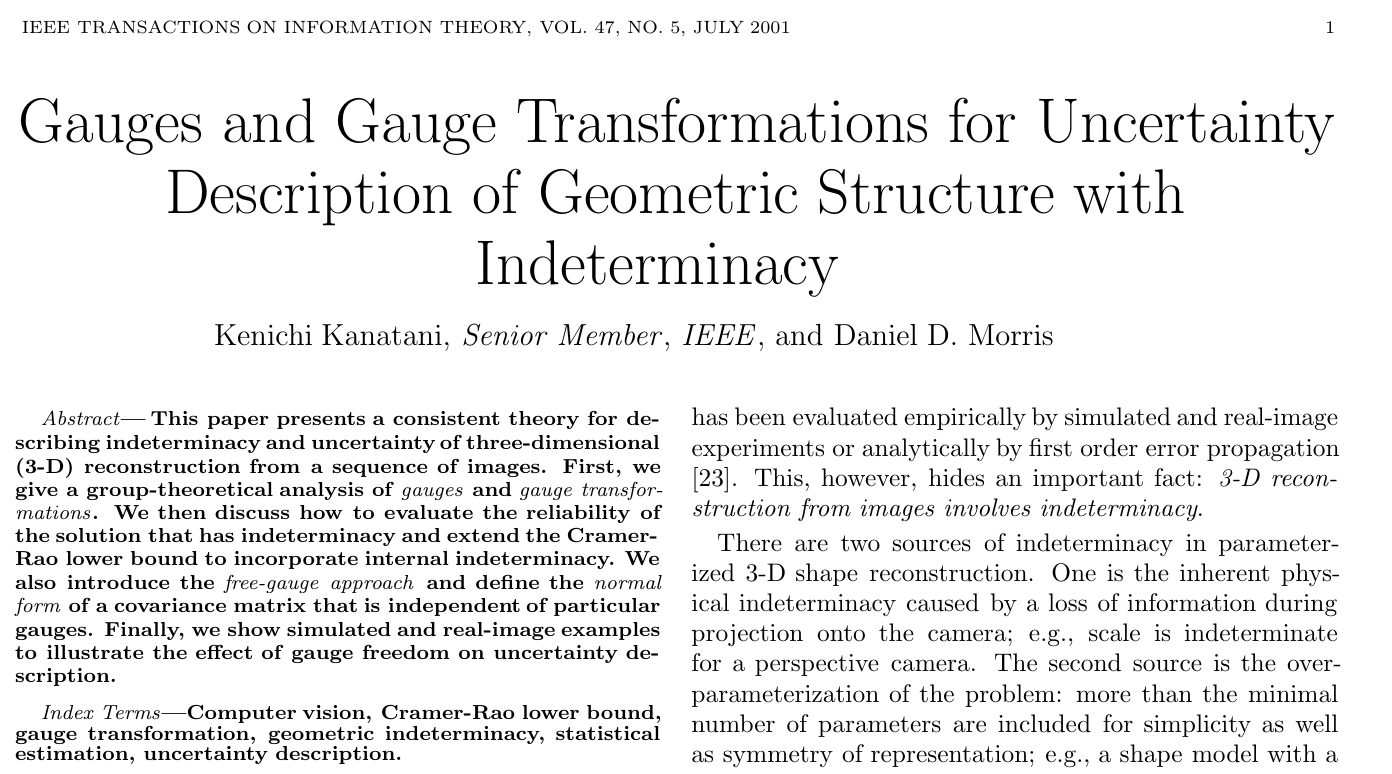# 3. 介绍

• 我们可以将坐标原点固定在对象的特定点上，并将对象的大小标准化为单位长度
• 而固定参数则定义为没有不确定性，其他所有参数的不确定性均被改变

# 4. 3D重建问题

## 4.1. 目标函数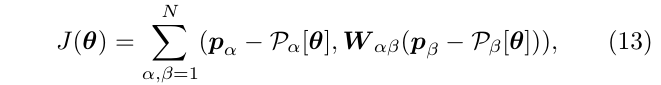# 5. Gauges

gauge freedom的存在表明了在参数空间$$\mathcal{M}$$中，存在一个光滑流形，对于任意的点$$\theta$$其给出的解都能使目标函数的值一样。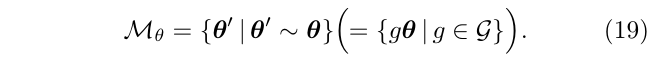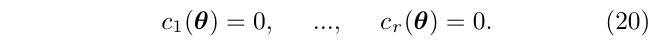• 它们在代数上是独立的，共同定义了一个在参数空间$$\mathcal{M}$$中的submanifold $$\mathcal{C}$$，称为gauge manifold
• gauge manifold $$\mathcal{C}$$与参数空间$$\mathcal{M}$$中的所有叶子，即与$$\mathcal{M}_\theta$$相交于一点
• 对于任何$$\theta \in \mathcal{M}_\theta$$$$\theta_C=C \cap \mathcal{M}_\theta$$，存在一个独特(仅有一个)的变换g()，使得$$\theta_C=g\theta$$

## 5.1. gauge fix approach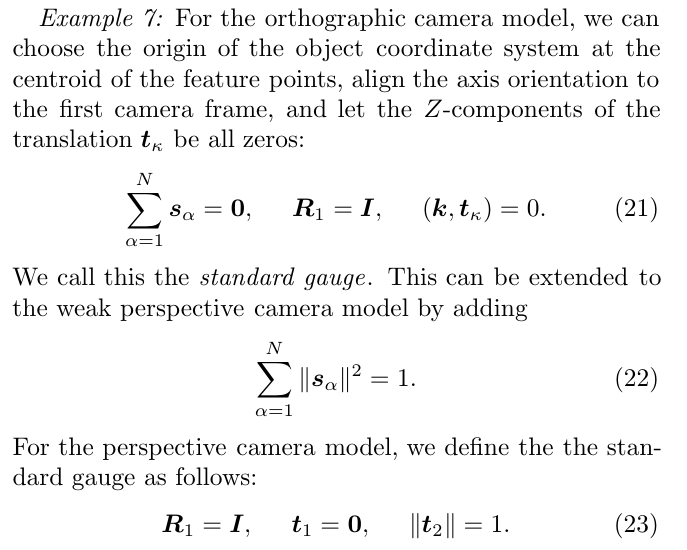• $$t_k$$是平移
• $$s_a$$是尺度

# 6. 估计的等价性定理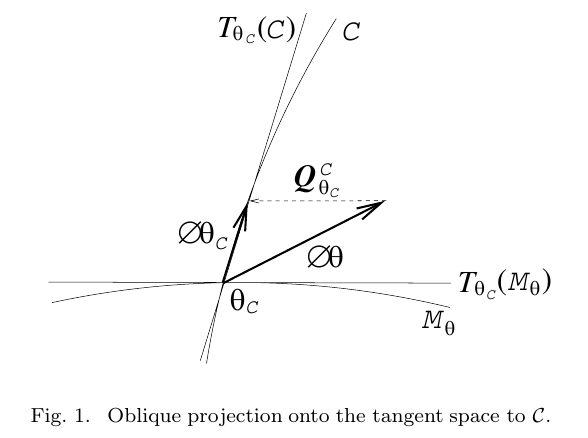• $$T_{\theta C}(\mathcal{M})$$是参数空间$$\mathcal{M}$$在参数$$\theta_C$$处的正切空间(tangent space)，是一个n维的线性空间
• $$T_{\theta C}(\mathcal{C})$$gauge manifold $$\mathcal{C}$$在参数$$\theta_C$$处的正切空间，它是$$T_{\theta C}(\mathcal{M})$$的(n-r)维子空间
• n: 参数向量$$\theta$$的维度数
• r: gauge freedom的自由度
• n-r: 可观测的维度

## 6.1. 图1的个人理解

• 已知在$$M_\theta$$轨道上，任意一点都可以满足使得目标函数$$J()$$最小化
• 假定轨道上某一点$$\theta_C$$处，求得了一个增量$$\phi_\theta$$，显然，这个增量有一部分分量不会改变目标函数$$J()$$的能量
• 在点$$\theta_C$$处，存在一个正切流形空间$$T_{\theta_C}(M_\theta)$$，增量$$\phi_\theta$$在这个正切空间上的投影量，只会使得$$\theta_C$$点沿着$$M_\theta$$轨道移动，而不是沿着垂直方向移动，因此，这部分分量并不改变目标函数$$J()$$的能量
• 那么这个在点$$\theta_C$$处的正切流形空间$$T_{\theta_C}(M_\theta)$$，也就是所说的零空间

## 6.2. 关于零空间的基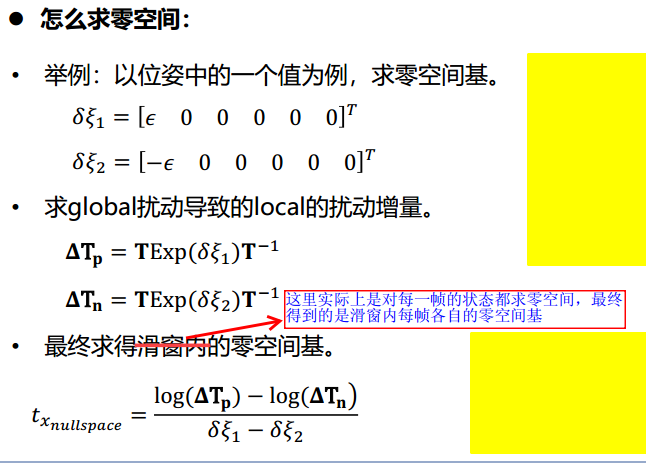## 6.3. 等价性的推导

$$\hat{\theta}_C$$是参数$$\theta$$对于gauge $$\mathcal{C}$$的估计。 使用一阶近似，那么作差$$\Delta \theta_C=\hat{\theta}_C-\theta_C$$可以使用$$T_{\theta C}(\mathcal{C})$$中的一个元素来标识

• 注意: $$\hat{\theta}_C$$$$\theta_C$$都表示状态
• $$\Delta \theta_C$$才表示增量

$$\Delta \theta \in T_{\theta C}(\mathcal{M})$$是一个任意的向量(也就是下图中的向量1)，则有:

• 当且仅当$$\Delta \theta-\Delta \theta_C \in T_{\theta C}(\mathcal{M}_\theta)$$ ，(也就是下图的向量1-向量2，得到向量的$$Q_{\theta_C}^C$$)，那么有:

$$\theta_C+\Delta \theta$$$$\hat{\theta}_C=\theta_C+\Delta \theta_C$$是几何等价的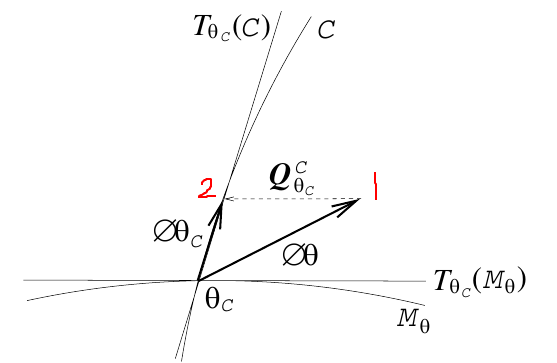$\Delta \theta_C=\Delta \theta + \sum_{i=1}^r x_i D_i(\theta_C)$

$\Delta \theta_C=\Delta \theta + U_{\theta_C} x \tag{36}$

• $$x=(x_1,\cdots,x_r)^T$$
• $$U_{\theta_C}=(D_1(\theta_C),\cdots,D_r(\theta_C))$$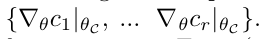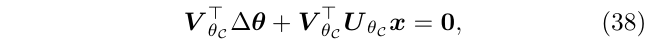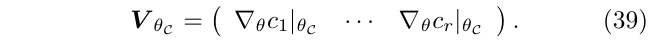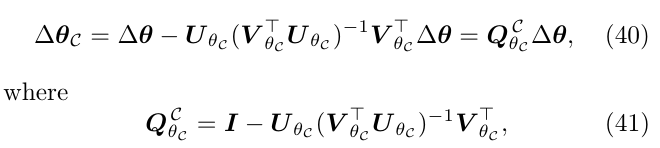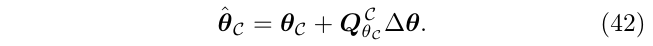# 7. 数值优化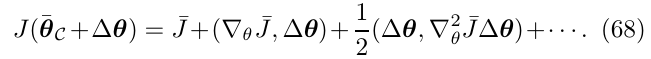• $$\triangledown_{\theta}J，\triangledown_{\theta}^2J$$分别表示目标函数$$J$$对参数$$\theta$$的一阶偏导和二阶偏导

• 表达式中符号上面的横杠，如$$\bar{J},\triangledown_\theta \bar{J}, \triangledown_\theta^2 \bar{J}$$表示的是在真值$$\bar{\theta}_C$$处进行计算得到的值（也就是线性化点得到值）

• $$\Delta \theta$$是使用真值$$\bar{\theta}_C$$处的正切空间$$T_{\bar{\theta}_C(\mathcal{M})}$$的元素来标识的

$$\Delta \theta$$是在$$T_{\bar{\theta}_C(\mathcal{M})}$$的局部坐标系下的，如 局部扰动向量$$\Delta R_{3\times1}$$ (回想四元数的更新，局部扰动)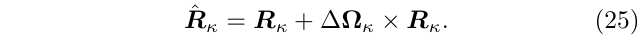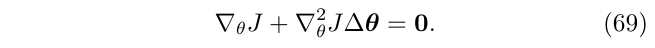\begin{aligned} H\Delta x=b \\ J^T J \Delta x = -J^T r \end{aligned}

1. 一是通过使用$$r$$条等式与(式69)进行结合（这相当于在目标函数中添加约束项(惩罚项)，作为先验）: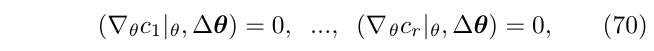1. 另外一种方法是，首先直接计算(式69)的任意一个解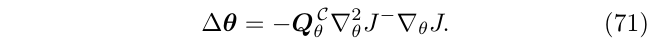(这个的意思是，先求出一个任意解，然后再减去这个解在零空间上的分量)

Free-Gauge Approach过程可以使用图来描述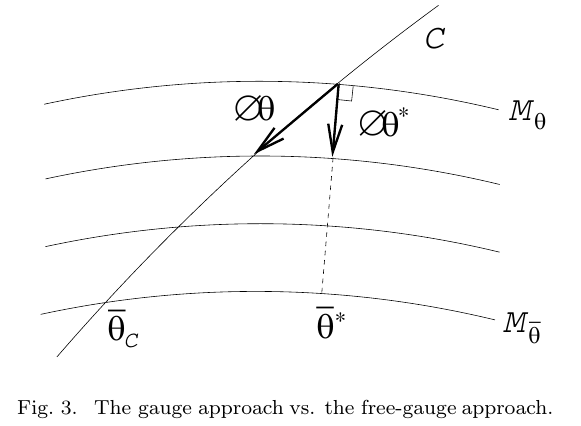• Free-Gauge Approach是沿着与$$\mathcal{M}_\theta$$正交的方向去迭代的，因此，其收敛速度会更快一些。